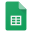# Waves

## Topic Summary

• The properties of transverse and longitudinal Waves
• The properties of Electromagnetic waves
• Applications of Electromagnetic waves

### Transverse and Longitudinal waves

Transverse waves: Particles move perpendicular to direction of motion of wave

Example: Water waves

Longitudinal waves: Particles move along direction of motion of wave

Example: Sound waves### The Electromagnetic SpectrumWaves Exam question 2 (from AQA Paper 6H Specimen)

## Observing properties of waves in a ripple tank (Required Practical)

Waves in a fluid can be observed using a ripple tank.

There are various ways that this can be done:

• The frequency of a wave can be found by counting the number of waves that pass a point every second
• The wave speed could be calculated by timing how long it takes a wave to travel a fixed distance.
• The wavelength can either be observed by using a metre rule or could be calculated once the wave speed and frequency is known.

Improving quality of results:

• Measure the wavelength of 10 waves and then divide length by 10
• Measure the time taken for 10 waves to pass a fixed point and divide by 10
• Take repeat measurements
• Ensure the lab is darkened so that wave fronts can be observed more clearlyWaves Checklist